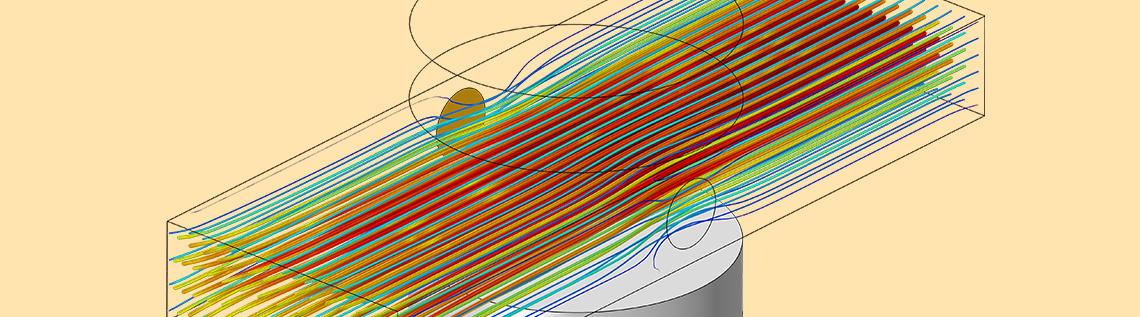# 在 COMSOL® 中构建磁流体动力学多物理场模型

2019年 6月 19日

COMSOL Multiphysics® 软件中的模型都是从零开始构建的，软件支持多物理场，因此用户可以按照自己的意愿轻松地组合代表不同物理场现象的模型。有时这可以通过使用软件的内置功能来实现，但有些情况下，用户需要做一些额外的工作。我们以构建磁流体动力学（MHD）模型为例介绍一下这个工作流程。

### 磁流体动力学的多物理场建模

MHD 现象的建模本质上是一个多物理场问题；必须用数值方法求解流体流动、电流和磁场之间的耦合问题。这些不同的场都是由偏微分方程描述的，可以通过有限元方法求解。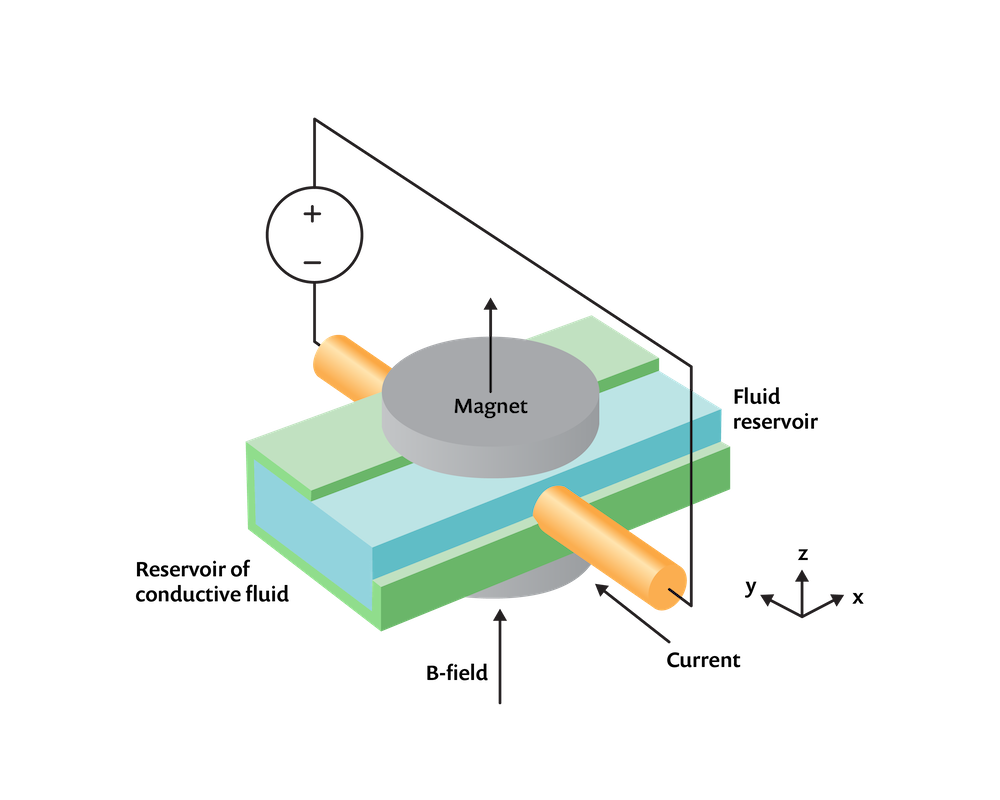\mathbf{J} = \sigma \left( – \nabla V + \mathbf{v \times B}\right)

#### 耦合电场、磁场和流场

\nabla \times \left( \mu_0^{-1} \mu_r^{-1} \mathbf{B} \right) = \mathbf{J}

\nabla \cdot \mathbf{J} = 0

\nabla \times \left( \mu_0^{-1} \mu_r^{-1} \mathbf{(B+B_r)} \right) = \mathbf{0}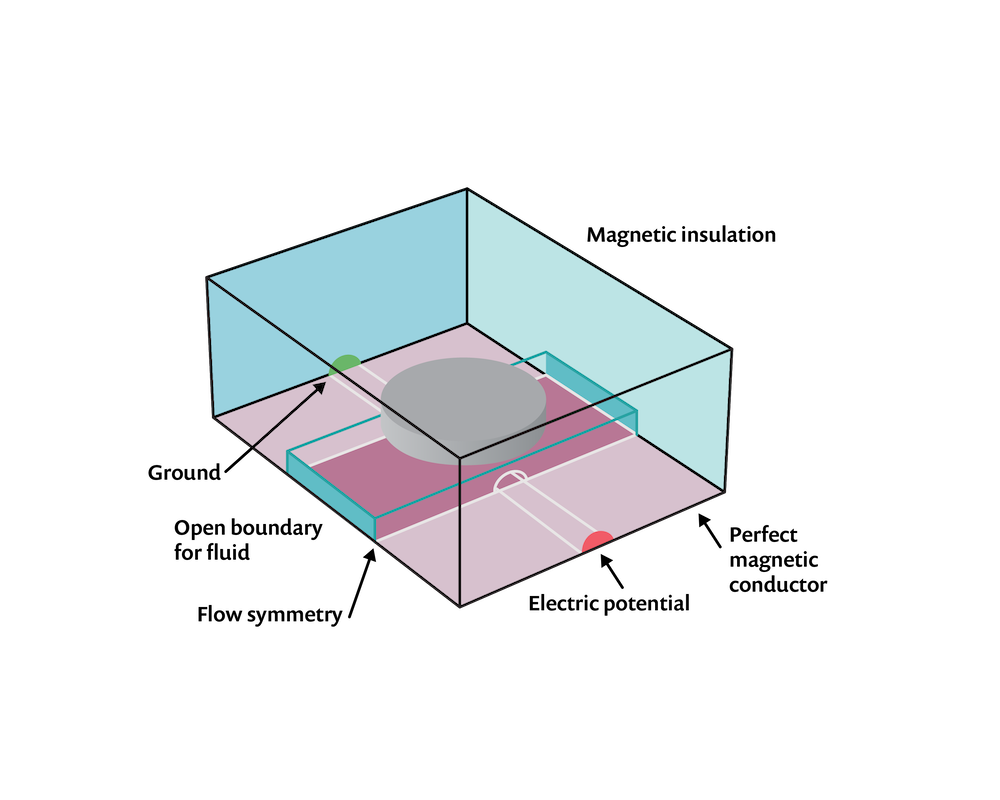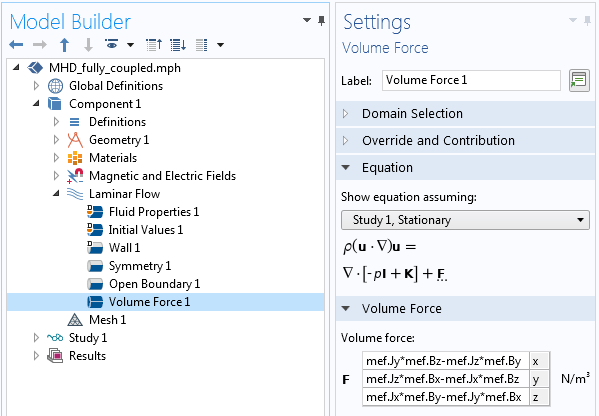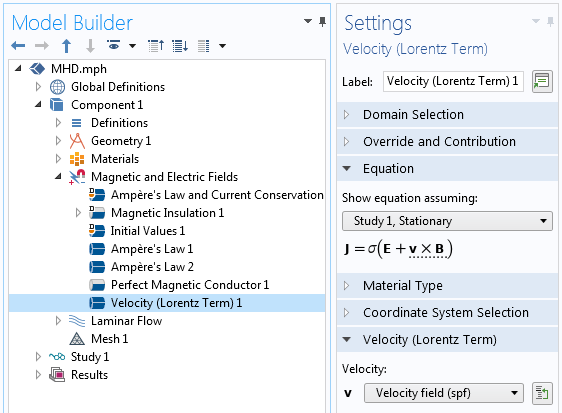#### MHD 问题的网格划分和求解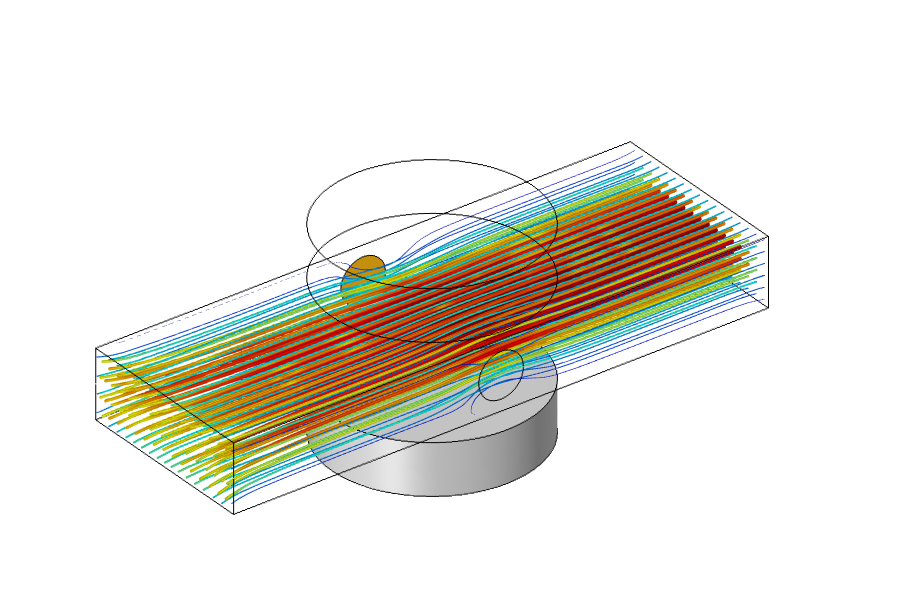### 简化 MHD 模型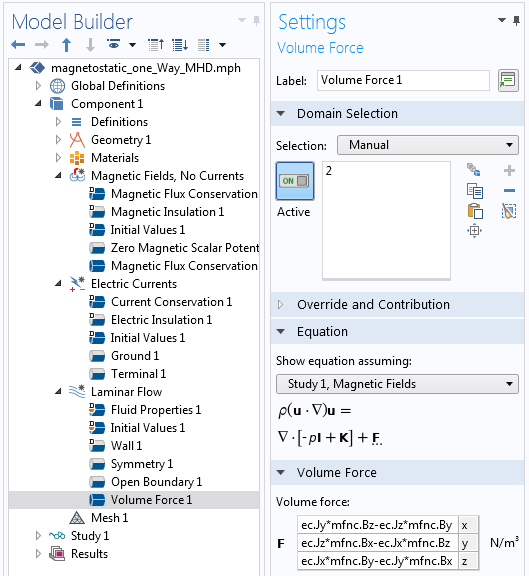#### 评论 (1)

##### 留言2019-09-26